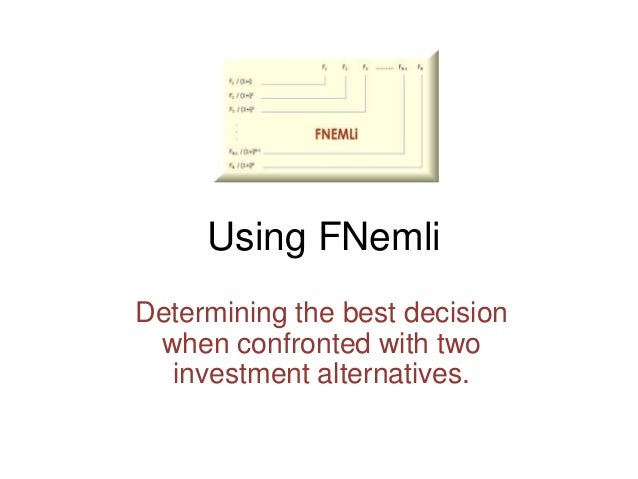Successfully reported this slideshow.Upcoming SlideShare
×

# Mathematics of Finance with a Smile.

651 views

Published on

Learning / Teaching Financial Math via Quantitative Interactive Workbook Courseware
QIWCourseware by Dan DuPort

• Full Name
Comment goes here.

Are you sure you want to Yes No• Be the first to comment

• Be the first to like this

### Mathematics of Finance with a Smile.

1. 1. Using FNemli Determining the best decision when confronted with two investment alternatives.
2. 2. Using FNemli • The following (4 slides) illustrates how beginning finance students can learn about using value in decision making. How would you tell someone about the equal periodic amount you can withdraw from a saving account and be left with 0 after N withdrawals? • Most beginning mathematics of finance texts simply state or derive formulas and then drill students on solving for the various variables given the values of the others in the formula. But the real heart of the mathematics of finance is the use of the concepts to provide insight into making value judgments.
3. 3. Given that I can receive an amount in the future, FV, then the value of that amount today being worth PV = FV / (1+i)N is a fundamental principle of Finance. It stems from the idea the PV can be invested in a fund with compound interest i, for N periods to achieve the amount FV. I emphasis the word "can" and the idea of "in principle". The value of the amount in the future is independent of what is actually done with the money that you get along the way. So even though the actual amounts, P, may not be invested, the value of the receiving of them is still given by the future value formula for FV. A regular annuity's future value with payment P, interest i per period, for N periods is: FV = P [ (1+i)0 + (1+i)1 + (1+i)2 + …. + (1+i)N-1 ] = P [ (1+i)N – 1 ] / i
4. 4. When a loan of amount A is taken out by an individual or a commercial business, an interest rate, i, and a term, N, are what determines the payment amount, P. As we know the Payment P must be such that A = the present value of the future value, FV, of the annuity created by P. FV = P [ (1+i)N – 1 ] / i , as announced on the previous page. Its present value, PV, is FV / (1+i)N ={P [ (1+i)N – 1 ] / i }/ (1+i)N = P [ 1 - (1+i)-N ] / i Why is that? The lender of the loan has two choices. The lender can, instead of making the loan, invest the loan amount at the rate i compounded for N periods. But if the loan is made, the lender can reinvest each payment as received into an annuity of interest rate i, for N periods, to get the same future value.
5. 5. For example, to make this really clear, if you were to loan a friend 1000 dollars, and the friend was trustworthy in that the payments would be made on time, then instead leaving the 1000 in a bank account and obtaining compound interest at i% per period for N periods, you could give your friend the 1000 dollars and have your friend pay you N equal payments of an amount P, deposit each in an annuity when received, and after N periods, you would have 1000(1+i)N because FV = P [ (1+i)N – 1 ] / i = ( P [ 1 - (1+i)-N ] / i ) (1+i)N = PV (1+i)N = 1000(1+i)N You have lost nothing by making this loan to your friend. This illustrates the fundamental rule of equalization in finance: If I have A (dollars) and if I can get Ã one way, and get the same Ã a different way, then there is no choice to make between the two if the risk involved in getting Ã is the same in both cases.
6. 6. It is just this principle that allows you to make finance decisions and also to solve financial problems. How can I use this equalization rule to determine the amount of money, A, I need today to be able to draw an amount P from it every period for N periods if the assumed interest rate is i per period? We argue as before: If I have A today, then in N periods I can obtain A (1+i)N by placing A in the bank. On the other hand, if I get P every period, for N periods, I can build an annuity of N payments with future value FV = P [ (1+i)N – 1 ] / i . After the N withdrawals, the two must be equal. So A (1+i)N = P [ (1+i)N – 1 ] / i. And therefore A = P [ 1 - (1+i)-N ] / i is simply the present value of the annuity that I would get by depositing the P each period into an account, even though I might not do that.
7. 7. Using FNemli Download a copy of FNemli from QIWCourseware.com Use it for free, no strings attached, for learning the basics of Mathematics of Finance. 28 pages of interactive, visual learning that’s easy on the mind – from TVM to MIRR. Requires Excel 2010 for the PC Excel 2011 for the MAC or higher